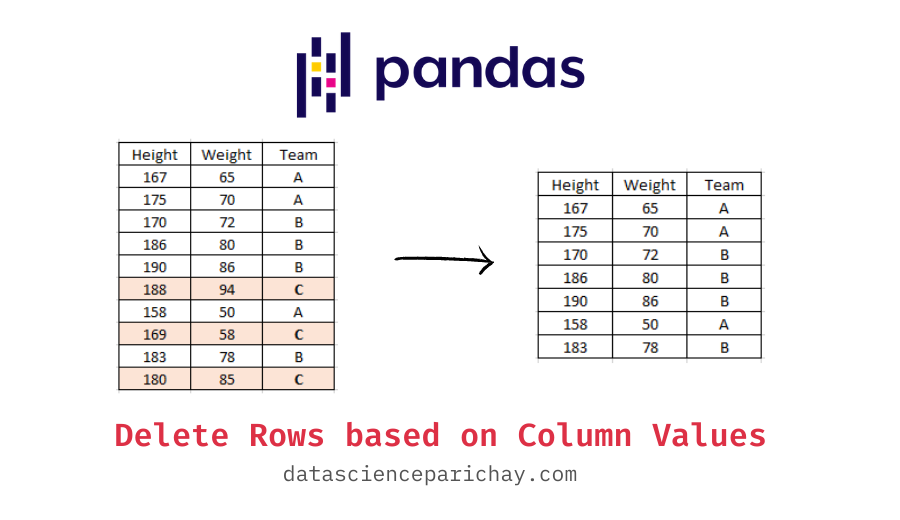# Pandas – Delete rows based on column values

Pandas is a powerful library for manipulating tabular data in python. When working with pandas dataframes, it might happen that you require to delete rows where a column has a specific value. In this tutorial, we will look at how to delete rows based on the column values of a pandas dataframe.

There are a number of ways to delete rows based on column values. You can filter out those rows or use the pandas dataframe `drop()` function to remove them. The following is the syntax:

```# Method 1 - Filter dataframe
df = df[df['Col1'] == 0]
# Method 2 - Using the drop() function
df.drop(df.index[df['Col1'] == 0], inplace=True)```

Note that in the above syntax, we want to remove all the rows from the dataframe df for which the value of the “Col1” column is 0.

Let’s look at these methods with the help of some examples. First, we will create a sample dataframe that we’ll be using to demonstrate the different methods.

```import pandas as pd

# dataframe of height and weight football players
df = pd.DataFrame({
'Height': [167, 175, 170, 186, 190, 188, 158, 169, 183, 180],
'Weight': [65, 70, 72, 80, 86, 94, 50, 58, 78, 85],
'Team': ['A', 'A', 'B', 'B', 'B', 'C', 'A', 'C', 'B', 'C']
})

# display the dataframe
df```

Output:

The above dataframe contains the height (in cm) and weight (in kg) data of football players from three teams – A, B, and C.

To delete rows based on column values, you can simply filter out those rows using boolean conditioning. For example, let’s remove all the players from team C in the above dataframe. That is all the rows in the dataframe df where the value of column “Team” is “C”.

```# remove rows by filtering
df = df[df['Team'] != 'C']
# display the dataframe
df```

Output:

📚 Data Science Programs By Skill Level

Introductory

Intermediate ⭐⭐⭐

🔎 Find Data Science Programs 👨‍💻 111,889 already enrolled

Disclaimer: Data Science Parichay is reader supported. When you purchase a course through a link on this site, we may earn a small commission at no additional cost to you. Earned commissions help support this website and its team of writers.

You can see that all the rows where the value of column “Team” was “C” have been removed. Also, notice that the filtered dataframe retains the indexes from the original dataframe.

You can also use the pandas dataframe `drop()` function to delete rows based on column values. In this method, we first find the indexes of the rows we want to remove (using boolean conditioning) and then pass them to the drop() function.

For example, let’s remove the rows where the value of column “Team” is “C” using the drop() function.

```# remove rows using the drop() function
df.drop(df.index[df['Team'] == 'C'], inplace=True)
# display the dataframe
df```

Output:

You can see that all the rows with “C” as the value for the column “Team” have been removed. Again, notice that the resulting dataframe retains the original indexes. If you want to reset the index, use the pandas reset_index() function.

Also, notice that the condition used in this example is `df['Team'] == 'C'` because we want to know the indexes of the rows to drop. In the previous example, we used the condition `df['Team'] != 'C'` because we wanted to know the indexes of the rows to keep post-filtering.

For more on the pandas drop() function, refer to its documentation.

With this, we come to the end of this tutorial. The code examples and results presented in this tutorial have been implemented in a Jupyter Notebook with a python (version 3.8.3) kernel having pandas version 1.0.5

•